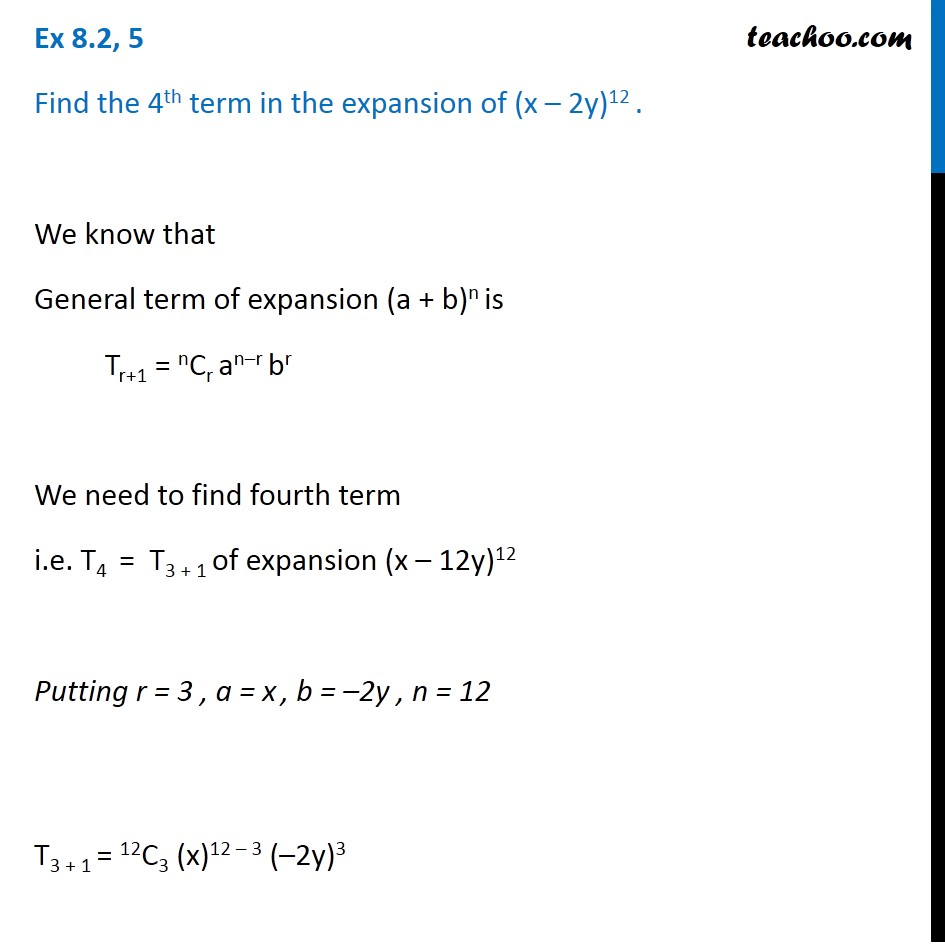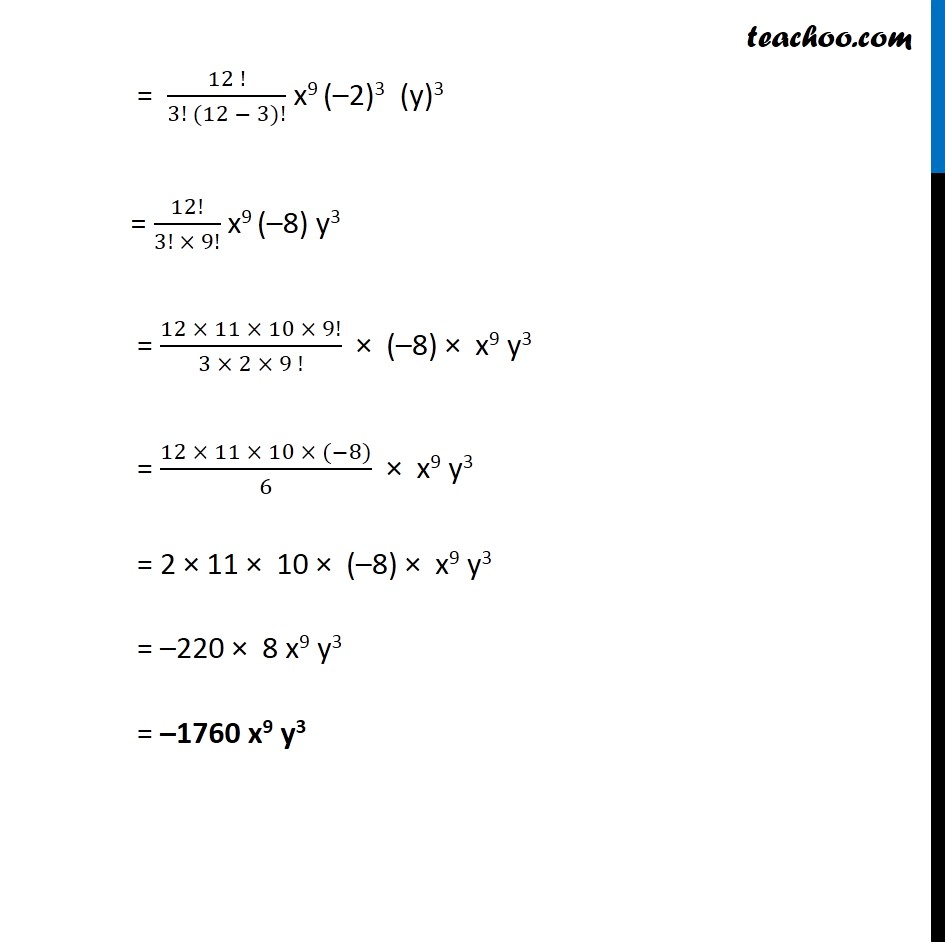1. Chapter 8 Class 11 Binomial Theorem (Deleted)
2. Concept wise
3. Coefficient

Transcript

Ex 8.2, 5 Find the 4th term in the expansion of (x – 2y)12 . We know that General term of expansion (a + b)n is Tr+1 = nCr an–r br We need to find fourth term i.e. T4 = T3 + 1 of expansion (x – 12y)12 Putting r = 3 , a = x , b = –2y , n = 12 T3 + 1 = 12C3 (x)12 – 3 (–2y)3 = (12 !)/(3! (12 − 3)!) x9 (–2)3 (y)3 = 12!/(3! × 9!) x9 (–8) y3 = (12 × 11 × 10 × 9!)/(3 × 2 × 9 !) × (–8) × x9 y3 = (12 × 11 × 10 × (−8))/6 × x9 y3 = 2 × 11 × 10 × (–8) × x9 y3 = –220 × 8 x9 y3 = –1760 x9 y3